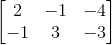Varsity Tutors always has a different Finite Mathematics Question of the Day ready at your disposal! If you’re just looking to get a quick review into your busy day, our Finite Mathematics Question of the Day is the perfect option. Answer enough of our Finite Mathematics Question of the Day problems and you’ll be ready to ace the next test. Check out what today’s Finite Mathematics Question of the Day is below.

### Question of the Day: Finite Mathematics

Consider the following payoff matrix for a game:Give the value of the saddle point entry of the matrix, if it exists.The matrix has no saddle point.

### All Finite Mathematics Resources